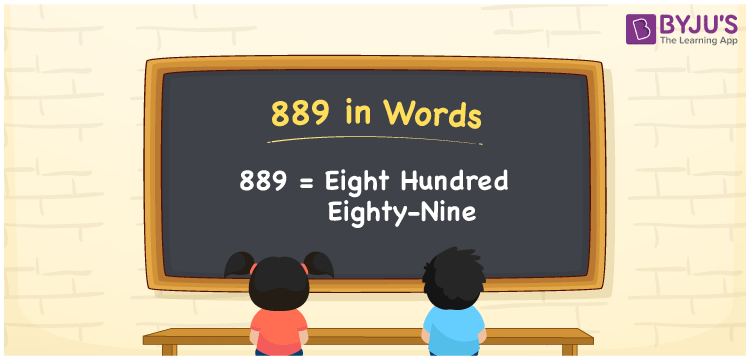# 889 in words

889 in words is written as Eight Hundred and Eighty Nine. 889 represents the count or value. The article on Counting Numbers can give you an idea about count or counting. The number 889 is a 3 digit number that is used in expressions related to money, days, distance, length, weight and so on. Let us consider an example for 889. “The oil packet had Eight Hundred and Eighty Nine milliliters of oil instead of one liter.”

 889 in words Eight Hundred and Eighty Nine Eight Hundred and Eighty Nine in Numbers 889

## 889 in English Words## How to Write 889 in Words?

We can convert 889 to words using a place value chart. The number 889 has 3 digits, so let’s make a chart that shows the place value up to 3 digits.

 Hundreds Tens Ones 8 8 9

Thus, we can write the expanded form as:

8 × Hundred + 8 × Ten + 9 × One

= 8 × 100 + 8 × 10 + 9 × 1

= 889

= Eight Hundred and Eighty Nine.

889 is the natural number that is succeeded by 888 and preceded by 890.

889 in words – Eight Hundred and Eighty Nine.

Is 889 an odd number? – Yes.

Is 889 an even number? – No.

Is 889 a perfect square number? – No.

Is 889 a perfect cube number? – No.

Is 889 a prime number? – No.

Is 889 a composite number? – Yes.

## Solved Example

1. Write the number 889 in expanded form

Solution: 8 × 100 + 8 × 10 + 9 × 1

We can write 889 = 800 + 80 + 9

= 8 × 100 + 8 × 10 + 9 × 1.

## Frequently Asked Questions on 889 in words

Q1

### How to write the number 889 in words?

889 in words is written as Eight Hundred and Eighty Nine.
Q2

### Is 889 divisible by 7?

Yes. 889 is divisible by 7.
Q3

### Is 889 a prime number?

No. 889 is not a prime number.